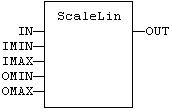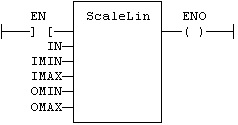# ScaleLin

Function - Scaling - linear conversion.

## Inputs

IN   : REAL  Real value
IMIN : REAL  Minimum input value
IMAX : REAL  Maximum input value
OMIN : REAL  Minimum output value
OMAX : REAL  Maximum output value

## Outputs

OUT : REAL   Result: OMIN + IN * (OMAX - OMIN) / (IMAX - IMIN)

## Truth table

 inputs OUT IMIN >= IMAX = IN IN < IMIN = IMIN IN > IMAX = IMAX other = OMIN + IN * (OMAX - OMIN) / (IMAX - IMIN)

## Remarks

In LD language, the operation is executed only if the input rung (EN) is TRUE. The output rung (ENO) keeps the same value as the input rung. In IL, the input must be loaded in the current result before calling the function.

## ST Language

OUT := ScaleLin (IN, IMIN, IMAX, OMIN, OMAX);

## FBD Language## LD Language

(* The function is executed only if EN is TRUE *)
(* ENO keeps the same value as EN *)## IL Language

Op1: LD       IN
ScaleLin IMAX, IMIN, OMAX, OMIN
ST       OUT